Time: 3 Hours                                                                                                     Max. Marks: 100

NOTE: There are 9 Questions in all.

·      Question 1 is compulsory and carries 20 marks. Answer to Q. 1. must be written in the space provided for it in the answer book supplied and nowhere else.

·      Out of the remaining EIGHT Questions answer any FIVE Questions. Each question carries 16 marks.

·      Any required data not explicitly given, may be suitably assumed and stated.

Q.1       Choose the correct or best alternative in the following:                                         (2x10)

a.       A JK flip-flop can be implemented using D flip-flop connected such that

(A)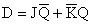(B)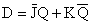(C)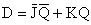(D)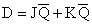b.      An effective solution to the power consumption problem lies in using _________ transistors to implement ICs.

(A) NMOS                                         (B)  TTL shottky

(C) PMOS                                          (D)  both NMOS & PMOS

c.   Memory interleaving technique is used to address the memory modules in order to have

(A)    higher average utilization

(C)    reduced complexity in mapping hardware

(D)    both (A) & (B)

d.   In a multiprogramming system, which of the followings is used.

(A)    Data parallelism                           (B)  Paging concept

(C)  L1 cache                                     (D)  None of the above

e.   Cycle stealing technique is used in

(A)     Interrupt based data transfer         (B)  Polled mode data transfer

(C)  DMA based data transfer             (D)  None of these

f.    Manipulation of individual bits of a word is often referred to as

(A)     Bit twiddling                                 (B)  Bit swapping

(C)  Micro-operation                           (D)  None of these

g.   Which of the following is not a characteristic of a RISC architecture.

(A)     Large instruction set                     (B)  One instruction per cycle

(C)  Simple addressing modes              (D)  Register-to-register operation

h.   When CPU is not fully loaded, which of the following method of data transfer is preferred

(A)    DMA                                           (B)  Interrupt

(C)  Polling                                          (D)  None of these

i.    Associative memory is some times called as

(A)   Virtual memory                             (B) Cache memory

(C) Main memory                                (D) Content addressable memory

j.    BCD equivalent of Two’s complement is

(A)    nine’s complement                        (B)  ten’s complement

(C)  one’s complement + 1                  (D)  none of these

Answer any FIVE Questions out of EIGHT Questions.

Each question carries 16 marks.

Q.2     a.   Show the Truth Table’s for the following functions:

(i)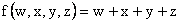(ii)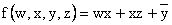(2 + 3)

b.   Construct a T flip-flop using a

(i)  D-FF                                                   (ii)  J-K FF                                             (5)

c.   Explain Von Neumann architecture and stored program concept.                        (6)

Q.3     a.   Show the hardware to implement the following micro-operations.

(i)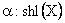(ii)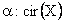‘X’ consist of four D-FFs.                                                                                  (6)

b.   Discuss the properties of an ideal instruction set computer.                                  (4)

c.   Explain instruction cycle.  Implement the RTLs of fetch phase.                             (6)

Q.4     a.   Design a 2-bit adder and logic circuit capable of performing AND, ADD, complement, and shift left operation.                                            (8)

b.   Discuss the different addressing modes of an instruction.                                     (8)

Q.5     a.   What is the significance of program status word.                                                 (4)

b.   What is software interrupt?  State its use.                                                            (5)

c.   What do you mean by instruction pipelining.  Discuss different methods used to overcome the major difficulties that cause the instruction pipeline to deviate from its normal operation.                (7)

Q.6             Design a control unit (Micro programmed) to implement the following instructions:-

 Instruction Instruction Code Operation AND 00 AAAAAA AC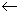AC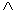M[AAAAAA] JMP 01 AAAAAA PCPC + AAAAAA ADD 10 AAAAAA ACAC + M[AAAAAA] REV 11 XXXXXX AC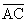Give your own specification and design considerations.                                     (16)

Q.7     a.   What is the difference between 1’s complement subtraction and 2’s complement subtraction of binary numbers?  Show it by example.            (6)

b.   Show the step-by-step multiplication process using Booth’s algorithm, when +14 is multiplied by –14.  Assume 5-bit registers that hold signed numbers.                                                                (10)

Q.8     a.   Compare I/O versus memory bus.                                                                      (2)

b.   Explain the use of time out mechanism in hand shaking data transfer scheme.                       (2)

c.   Explain virtual memory & its mapping schemes.                                                (10)

d.   State the advantages of cache memory.                                                              (2)

Q.9     a.   With neat block diagram, explain the working principle of micro program sequencer.                      (7)

b.   Compare memory mapped I/O vs I/O mapped I/O.                                           (5)

c.   Give the flow chart for multiplication of two floating-point numbers.                     (4)